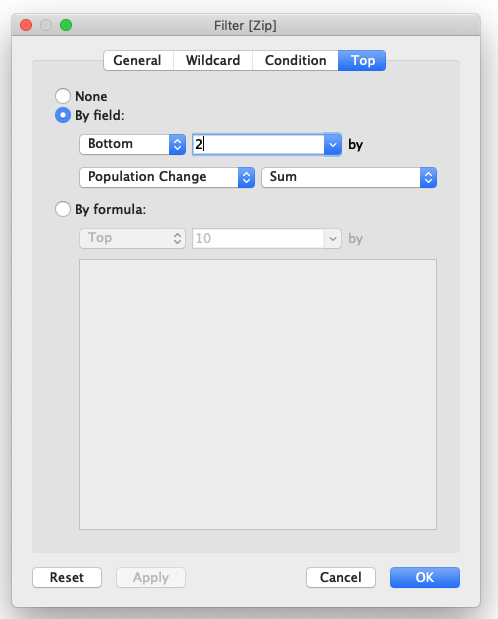2 views
in BIHi, I am new to tableau and working on a project:

I have created a dumbell chart viz where the total population is displayed between 2 years 2014 and 2017 for about 70 zip codes.

I want to add a filter such that only the top 10 zip codes are shown which have the greatest decrease in population 2014-2017.

Will a calculated field be required for this? Or is there a simple way to just filter?

For example, I have filtered by Top 10 - variance but it is showing me incorrect figures

by (47.2k points)
• Yes, you will need to use a calculated field and LOD (Level of Detail) expressions to determine the difference in population between the years.

• Create a calculated field under Measures called "Population Change". Use the following code:

{ FIXED [Zip] : SUM(IIF(YEAR([Year]) = 2017, [Total Population], NULL)) } - { FIXED [Zip] : SUM(IIF(YEAR([Year]) = 2014, [Total Population], NULL)) }

• Drag Zip onto Filters. In the window that comes up, select the "Top" tab, then the "By Field" radio button, then "Bottom 10 by Population Change Sum"• Going forward, if you have more than just 2014 and 2017's data, you can create a parameter for start year and end year then use those in the calculated field instead of hard coding them.